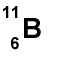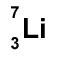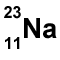MainTutorialsOrganic ChemistryPractice TestsOnline QuizzesReference ToolsBasic Practices:

(1) Instructions:
Tell how many protons, neutrons, and electrons each element has based on the information given.

 a.)b.)c.)d.)e.)(2) Instructions:
Name these compounds.

a.) CuCl b.) NaF c.) MnO2 d.) FeBr2 e.) Fe2O3

(3) Instructions:
Find the formula for each chemical below.

a.) Sodium sulfate   b.) Vanadium (V) fluoride    c.) Mercury (I) chloride   d.) Tin (II) fluoride   e.) Cobalt (III) nitrate

1. The atomic weight is 16 amu. Since we know that oxygen, with a 8, has 8 protons (that is the definition of a an atomic number), we can then deduce that oxygen has 8 neutrons (16-8 = 8). Final answer? 8 protons, 8 electrons, and 8 neutrons. Please note that in an elemental state, the number of electrons and protons will always be equal.
2. With an atomic weight of 11 amu, along with an atomic number of six, boron has 5 neutrons. (11 amu - 6 protons = 5 neutrons)
3. 4 protons, 4 electrons, and 5 neutrons
4. 3 protons, 3 electrons, and 4 neutrons
5. 11 protons, 11 electrons, 12 neutrons

1. By the rules, this is Copper Chloride. But copper can have different charges, so you must figure what the charge on this one is. Let's see: you know chloride can have only one value, and that's a -1. So if they must add up to zero, Cu must be +1. The answer is copper (I) chloride.
2. This is sodium and fluorine, so the name is sodium fluoride. Sodium can only be +1, so there is no need for roman numerals.
3. The name is Manganese Oxide, but a roman numeral is necessary, since it's a transition metal. In this case, manganese is a transition metal, so it needs a roman numeral. Let's start with oxygen: Each oxide ion has a charge of -2, and there are 2 of them. So the total charge due to oxide is -4. The single manganese ion must compensate for this, so its charge must be +4. The name is manganese (IV) oxide.
4. Same here. It needs a roman numeral. Br is -1, and 2 of them, so Fe must be +2. Name is Iron (II) Bromide.
5. e.This one's a little bit different, but the same concepts apply. You start with the oxygens. 3 of -2 ions make it a total of -6 charge due to oxide. So the two irons must compensate. If two of them must equal +6, each must be...+3!!! It should be no surprise. So the name of this is Iron (III) Oxide.news | about us | contact us tutorials index | organic chemistry | practice tests | online quizzes | reference tools site copyright (c) 2002-2013 Learn ChemSubscribe to our low volume newsletter to receive up-to-date information about the CHEM SITE